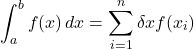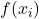One of the pivot points you might stumble upon in Calculus 2 class is Numerical Integration. It is often referred to as Approximation of Areas.

The idea is that the integral is replaced by a sum, where the integrand is sampled in a number of discrete points. One of the way to describe it isLeft side of equation : We know that integration between two points is actually the area under the curve and x axis (presuming we integrate with respect to x).

Right side of equation : Another way to find area is be to break the complete region into smaller pieces. Simplest way is to take these pieces as rectangles. Area of one rectangle would be length x Width. Length(or height) is represented asand width is resented as. This is the story of only one such rectangle, but if we have broken the region in n such rectangles, then we need to add all such areas. That’s what summation is doing (with upper limit n)

Above method is called Reimann’s sum.

A follow-up question which you might have is, how do we choose i in. We have three ways to do it : Left end point, Right end point and Mid point. I have provided a detailed explanation and examples in the video below.

Why is this approximation? Integration can be visualized as breaking the area into infinite regions and summing up the individual areas. We can’t practically divide region into infinite rectangles. So we try to make as many regions as possible. The more regions there are, the closer the approximation will be.

Why approximate when we can have actual? Often, the mathematical function f(x) to be integrated is too complex and we are not able to integrate it analytically. Or, in some cases, the function is one that has been obtained from experimental data, and no mathematical equation is available to represent the data so that they can be integrated analytically. In these cases, we can use numerical integration.

Is rectangle the only shape in which we break? No really. It can be trapezium (called trapezoidal rule), it can also be quadratic approximation (called Simpson’s rule)

Which one is most accurate? Among Reimann’s, Trapezoidal and Simpson’s, Simpson’s wins the race of being more accurate. Quadratic approximation is always more accurate than linear approximation.